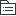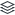# 3.消化系统疾病

151
|
32

## 相关模板推荐

•## 3.消化系统疾病 —— 作品大纲

•胃食管反流病
• 诊断公式
• 反酸+胸骨后烧灼感+胃镜检查食管下段红色条样糜烂带（烧心、反酸、返食）
• 原则
• LES功能障碍
• 使得LES受损的因素
• 手术后
• 腹内压增高
• 胃内压
• LES功能障碍
• 导致LES一过性松弛
• 激素、
• 食物
• 药物
• CCB，镇静剂
• 食管的清除作用降低
• 容量清除
• 食管粘膜的屏障功能降低
• 诊断依据
• 鉴别诊断
• 炎症
• 特殊疾病
• 冠心病
• 消化性溃疡
• 肿瘤
• 食管肿瘤
• 进一步检查
• 检验科
• 血脂，血糖，心肌酶谱，肿瘤标记物
• 影像科
• 消化道吞钡检查
• 心电图，超声心动图，胸片
• 特殊检查
• 胃镜
• 上消化道钡剂造影检查
• 食管 pH 监测和食管压力测定，
• 治疗原则
• 对症治疗
• 一般治疗，适当控制饮食，没做有氧运动
• 药物治疗
• 抑酸药物治疗
• 促进胃肠动力剂
• 手术治疗
• 预防
• 治疗并发症 高血压治疗
•食管癌
• 诊断公式
• 进行性吞咽困难（中晚期）＋胸骨后烧灼样疼痛（早期）＋进食哽咽感（早期）
• 初步诊断
• 诊断依据
• 鉴别诊断
• 炎症
• ：食管炎，
• 特殊疾病
• 食管憩室，食管静脉曲张，
• 贲门失驰缓症
• 肿瘤
• 食管良性肿瘤，
• 进一步检查
• 检验科
• 影像科
• 胸部 CT，骨扫描，头 CT
• 特殊检查
• 胃镜检查和活检病理学检查
• X线钡餐
• 治疗原则
• 对症治疗
• 营养支持
• 药物治疗
• 酌情放化疗
• 手术治疗
• 开胸手术
• 预防
•胃炎
• 诊断公式
• 饮食不洁或刺激物/NSAIDs＋上腹痛、腹胀、恶心呕吐
• 初步诊断
• 急性胃炎
• 急性胃粘膜病变
• 慢性胃炎
• 慢性浅表性胃炎
• 慢性萎缩性胃炎
• 自身免疫性胃炎
• 诊断依据
• 鉴别诊断
• 炎症
• 急性胆囊炎，胆石症
• 急性胰腺炎，急性阑尾炎
• 特殊疾病
• 消化性溃疡
• 进一步检查
• 检验科
• 肝肾功能电解质
• 特殊检查
• 胃镜
• 与鉴别诊断相关
• 血尿淀粉酶，腹部B超
• 治疗原则
• 对症治疗
• 去除病因
• 药物治疗
• 抗酸剂
• 胃黏膜保护剂
• 手术治疗
• 预防
• 若有幽门螺旋杆菌，择期行抗幽门螺旋杆菌治疗
•消化性溃疡
• 诊断公式
• 初步诊断
• 胃溃疡
• 进食痛
• 十二指肠溃疡
• 饥饿痛
• 消化性溃疡版出血
• 消化性溃疡+呕血黑便
• 十二指肠溃疡并幽门梗阻
• 呕吐大量发酸的宿食+不含胆汁
• 诊断依据
• .周期性、节律性上腹痛 2.呕血、黑便，大便隐血阳性
• 3.查体上腹中压痛，四肢湿冷，脉压变小 4.Hb82g/L(
• 鉴别诊断
• 炎症
• 急性胃炎
• 胆囊炎
• 慢性胃炎
• 特殊疾病
• 胃溃疡
• 肿瘤
• 胃癌
• 进一步检查
• 检验科
• 肝肾功、电解质、动脉血气分析
• 影像科
• 腹部B超
• 特殊检查
• 胃镜
• 肿瘤编织物
• 幽门螺旋杆菌检测
• 治疗原则
• 对症治疗
• 胃肠减压，严禁饮食，休息
• 药物治疗
• 抑酸剂
• 手术治疗
• 胃大部切除术
• 预防
• 根除幽门螺旋杆菌
•消化道穿孔
• 诊断公式
• 子主题
• 溃疡病史＋突发上腹部剧痛＋腹膜刺激征＋膈下游离气体
• 初步诊断
• 急性弥漫性腹膜炎
• 体温升高，板状腹，
• 诊断依据
• 1.突然上腹部剧痛，伴腹膜刺激征 2.十二指肠溃疡病史
• 鉴别诊断
• 炎症
• 1.胆囊炎，胆囊结石急性发作
• 2.急性胰腺炎
• 3.急性胃肠炎
• 4.急性阑尾炎
• 进一步检查
• 检验科
• 影像科
• 立位腹部平片
• 腹部B超
• 特殊检查
• 诊断性腹腔穿刺
• 与鉴别诊断相关的检查
• 腹部CT或B超
• 治疗原则
• 对症治疗
• 禁食水，胃肠减压
• 药物治疗
• 抗生素
• 补液，维持电解质平衡
• 手术治疗
• 急诊手术
• 预防
•消化道出血
• 诊断公式
• 呕血/便血+失血性休克/慢性贫血表现+大便潜血实验阳性
• 初步诊断
• 诊断依据
• 鉴别诊断
• 炎症
• 急性胃炎
• 特殊疾病
• 十二指肠溃疡
• 胃底食管静脉曲张
• 肿瘤
• 胃癌
• 进一步检查
• 检验科
• 凝血四项
• 大便潜血
• 影像科
• B超
• 特殊检查
• 胃镜+活检
• 治疗原则
• 对症治疗
• 禁食水、休息
• 补液，支持疗法
• 药物治疗
• 抑酸剂：应用质子泵抑制剂
• 止血剂
• 应用胃黏膜保护剂
• 抗生素
• 手术治疗
• 胃镜下手术
• 预防
•胃癌
• 诊断公式
• 老年人＋黑便＋龛影＋慢性溃疡疼痛规律改变＋上腹痛＋腹部包块＋消瘦＋左锁骨
• 上淋巴结肿大
• 初步诊断
• 诊断依据
• 1.腹痛、食欲下降、乏力、消瘦
• 2.结膜苍白、剑突下深压痛
• 3.上消化道造影所见
• 4.便潜血2次(+)
• 鉴别诊断
• 炎症
• 胃炎
• 特殊疾病
• 胃溃疡
• 进一步检查
• 检验科
• 学肿瘤标志物
• 影像科
• 腹部CT
• 胸部X线片
• 特殊检查
• 胃镜+活检
• 治疗原则
• 对症治疗
• 补液、营养支持治疗
• 药物治疗
• 酌情化疗
• 手术治疗
• 酌情行胃癌根治术
• 预防
•肝硬化
• 诊断公式
• 肝炎病史＋门脉高压（脾大＋腹水＋蜘蛛痣）＋超声（肝脏缩小）
• 初步诊断
• 并发症
• 脾功能亢进
• 胃底食管静脉曲张
• 腹水
• 自发性腹膜炎
• 肝性脑病
• 诊断依据
• 鉴别诊断
• 炎症
• 肝炎
• 肿瘤
• 肝癌
• 有腹膜炎是
• 结核性腹膜炎
• 有腹水时
• 心肾原因所导致的腹水
• 进一步检查
• 检验科
• 肝肾功电解质
• 影像科
• 腹部B超+CT
• 特殊检查
• 肝炎病毒检查
• 胆红素检查
• 腹水常规、病原学及细胞学检查
• 治疗原则
• 对症治疗
• 营养支持
• 药物治疗
• 保肝药物
• 利尿药物
• 补充白蛋白
• 手术治疗
• 腹腔穿刺抽腹水
• 预防
• 酌情抗病毒治疗
•非酒精性脂肪性肝病
• 诊断公式
• 无饮酒史+肝功能异常（转氨酶ALT＞40U/L）
• 初步诊断
• 诊断依据
• 鉴别诊断
• 炎症
• 病毒性肝炎
• 特殊疾病
• 自身免疫性肝病
• 肿瘤
• 肝癌
• 进一步检查
• 检验科
• 肝肾功电解质
• 肝炎病毒标志物
• 鉴别诊断
• 自身免疫性肝病指标
• 特殊检查
• 治疗原则
• 对症治疗
• 饮食控制
• 药物治疗
• 手术治疗
• 预防
•肝癌
• 诊断公式
• 肝炎病史＋肝区疼痛＋AFP 升高＋肝大质硬＋腹水黄疸＋B 超占位
• 初步诊断
• 诊断依据
• 1.右上腹痛逐月加重，伴纳差，体重下降
• 2.乙型肝炎病史
• 3.巩膜轻度黄染， TBIL 上升， GGT 上升， A-FP 上升
• 4.B 超所见
• 鉴别诊断
• 炎症
• 病毒性肝炎
• 肝硬化
• 特殊疾病
• 肝囊肿
• 肿瘤
• 转移性肝癌
• 肝内其他占位病变
• 血管瘤，腺瘤
• 进一步检查
• 检验科
• 肝肾功电解质
• 肝炎病毒标志物
• 影像科
• 上消化道造影，钡灌肠检查
• CT
• 特殊检查
• 肝穿刺活检
• 治疗原则
• 对症治疗
• 休息，保肝、利尿、纠正低蛋白血症、对症治疗
• 药物治疗
• 手术治疗
• 预防
•腹部闭合性肾损伤
• 诊断公式
• 初步诊断
• 诊断依据
• 鉴别诊断
• 炎症
• 特殊疾病
• 肿瘤
• 进一步检查
• 检验科
• 影像科
• 特殊检查
• 治疗原则
• 对症治疗
• 药物治疗
• 手术治疗
• 预防
•胆石症
• 诊断公式
• 胆石症
• 阵发性右上腹绞痛＋莫非征阳性＋B 超强回声光团、声影
• 胆囊炎
• 阵发性右上腹绞痛＋莫非征阳性＋恶心呕吐+无黄疸
• 胆管炎
• 阵发性右上腹绞痛＋莫非征阳性＋恶心呕吐+黄疸
• 初步诊断
• 胆管炎
• 阵发性右上腹绞痛＋莫非征阳性＋恶心呕吐+黄疸
• 急性胆管炎
• 右上腹痛+寒战高热+黄疸
• 夏克三联征
• 急性梗阻性化脓性胆管炎AOSC
• 右上腹痛+寒战高热+黄疸+血压下降+神经精神症状
• 诊断依据
• 1.反复发作右上腹绞痛，近期出现 Charcot 三联征+血压下降+精神症状
• 2.DBIL(直胆)及 WBC 升高
• 3.有胆囊结石二次手术史
• 鉴别诊断
• 炎症
• 胆道损伤导致的狭窄、梗阻
• 特殊疾病
• 胆道蛔虫症
• 肿瘤
• 胆道下端肿瘤
• 进一步检查
• 检验科
• 尿常规，凝血功能检查
• 影像科
• 1.B 超、 CT
• 2.发作期避免应用 ERCP 或 PTC
• 3.MRCP
• 特殊检查
• 学肿瘤标志物
• 治疗原则
• 对症治疗
• 营养支持
• 药物治疗
• 抗感染措施
• 手术治疗
• .急诊开腹探查，总胆管探查，引流
• 预防
•急性胰腺炎
• 诊断公式
• 水肿型
• 暴饮暴食/慢性胆道病史＋持续上腹疼痛＋弯腰疼痛减轻＋淀粉酶检测
• 出血坏死性
• 水肿型症状＋腰腹部或脐周紫斑＋腹穿洗肉水样液体＋血糖高＋血钙低
• 初步诊断
• 出血坏死型：血尿淀粉酶值不一定高，有时反而会下降。确诊时选 CT
• 一周内测血淀粉酶，超过一周测脂肪酶
• 诊断依据
• 1. 急性上腹痛，向后腰背部放射，伴恶心呕吐，发烧
• 2.全腹肌紧张，压痛，反跳痛，有可疑腹水征
• 子主题
• 3.WBC 升高，血钙下降
• 4.影像学检查所见： B 超、腹平片
• 鉴别诊断
• 炎症
• 急性胆囊炎
• 特殊疾病
• 消化道穿孔
• 急性肠梗阻
• 肿瘤
• 子主题
• 进一步检查
• 检验科
• 肝肾功电解质（血钙）
• 影像科
• 腹部CT
• 腹部立位X线平片
• 特殊检查
• 1.血尿淀粉酶、腹水淀粉酶
• 治疗原则
• 对症治疗
• 禁食，胃肠减压
• 药物治疗
• 适当应用抗生素及生长抑素类制剂
• 抑制胰腺外分泌及胰酶活性
• 手术治疗
• 酌情考虑内镜治疗
• 酌情考虑外科治疗
• 预防
• 并发症
•溃疡性结肠炎
• 诊断公式
• 左下腹痛＋粘液脓血便＋（便意、便后缓解）＋抗生素无效
• 初步诊断
• 轻度
• 腹泻＜4次，
• 中度
• 重度
• 腹泻＞6次，明显粘液脓血便，体温＞37.5℃，脉率＞90次
• 诊断依据
• 鉴别诊断
• 炎症
• 克罗恩病
• 细菌性痢疾
• 特殊疾病
• 肠癌米吧病
• 结肠结核
• 肿瘤
• 结肠癌
• 进一步检查
• 检验科
• 肝肾功电解质、肿瘤标志物、
• 影像科
• X线钡餐
• 特殊检查
• 结肠镜+粘膜或组织病理学检查
• 治疗原则
• 对症治疗
• 休息，流质饮食，纠正水电质平衡紊乱，
• 药物治疗
• 免疫抑制剂
• 糖皮质激素
• 手术治疗
• 使病情变化，必要时手术
• 预防
•克罗恩病
• 诊断公式
• 右下腹痛+糊状便+无粘液脓血便+抗生素治疗无效
• 初步诊断
• 诊断依据
• 鉴别诊断
• 炎症
• 克罗恩病
• 细菌性痢疾
• 特殊疾病
• 肠癌米吧病
• 结肠结核
• 进一步检查
• 检验科
• 肝肾功电解质、肿瘤标志物、
• 影像科
• X线钡餐
• 特殊检查
• 结肠镜+粘膜或组织病理学检查
• 治疗原则
• 对症治疗
• 休息，流质饮食，纠正水电质平衡紊乱，
• 药物治疗
• 免疫抑制剂，5-氨基水杨酸制剂
• 糖皮质激素
• 手术治疗
• 使病情变化，必要时手术
• 预防
•肠梗阻
• 诊断公式
• 腹痛＋吐＋胀＋闭＋X 线（香蕉/液平）
• 初步诊断
• 病因：机械性和动力性
• 血运：单纯性和绞窄性
• 程度：完全性和不完全性
• 部位：高位和低位
• 诊断依据
• 1.急性阵发性腹痛，伴肠鸣音亢进
• 2.腹胀，呕吐；停止排便与排气
• 3.有腹部手术史
• 4.腹透有多个液平面
• 鉴别诊断
• 炎症
• 急性胃肠炎：有腹痛，呕吐，腹胀，但无腹泻
• 特殊疾病
• 输尿管结石：持续时间不会太长，尿(-)等
• 急腹症
• 消化道穿孔、胆囊炎等
• 进一步检查
• 检验科
• 1.尿常规及沉渣镜检
• 血酸碱度及电解质
• 影像科
• .B 超
• 特殊检查
• 治疗原则
• 对症治疗
• 禁食，胃肠减压，
• 药物治疗
• 抗生素
• 输液，纠正脱水及酸中毒
• 手术治疗
• 预防
•结肠癌
• 诊断公式
• 结肠癌
• 老年人＋消瘦＋排便习惯改变＋CEA＋腹部肿块
• 直肠癌
• 直肠刺激症状＋指诊带血＋脓血便＋消瘦＋大便变形
• 初步诊断
• 诊断依据
• 1.排便习惯改变，便次增加
• 2.暗红色血便，便潜血(+)
• 3.右下腹肿块
• 4.伴消瘦、乏力
• 鉴别诊断
• 炎症
• 炎症性肠病
• 特殊疾病
• 回盲部结核
• 阿米巴痢疾
• 肿瘤
• 进一步检查
• 检验科
• 肝肾功，电解质
• 影像科
• 腹部B超+CT
• 特殊检查
• 结肠镜
• 癌胚抗原
• 治疗原则
• 对症治疗
• 营养支持
• 药物治疗
• 化疗
• 手术治疗
• 肿瘤根治术
• 预防
•肠结核
• 诊断公式
• 结核＋腹部症状（腹痛、腹泻、右下腹部肿块）+回盲部激惹征
• 初步诊断
• 诊断依据
• 鉴别诊断
• 炎症
• 炎症性肠病
• 特殊疾病
• 肠寄生虫病
• 肿瘤
• 肠道肿瘤
• 进一步检查
• 检验科
• 肝肾功电解质
• 影像科
• 肠道X线钡餐检查
• 特殊检查
• PPD实验
• 结肠镜
• 粪便病原学检查
• 鉴别诊断
• 肿瘤标志物
• 治疗原则
• 对症治疗
• 休息与营养，
• 药物治疗
• 抗结核治疗：早期，联合，足量，足疗程
• 手术治疗
• 预防
•结核性腹膜炎
• 诊断公式
• 结核＋腹部炎症（腹痛、腹泻、腹壁柔韧感）
• 初步诊断
• 诊断依据
• 鉴别诊断
• 炎症
• 特殊疾病
• 肝硬化腹水
• 肿瘤
• 腹腔恶性肿瘤，
• 进一步检查
• 检验科
• 肝肾功电解质
• 影像科
• 腹部X线检查
• 特殊检查
• PPD试验
• 腹腔穿刺活检
• 腹腔镜
• 治疗原则
• 对症治疗
• 休息和营养
• 药物治疗
• 抗结核治疗
• 早期，规律，全程，适量，联合
• 手术治疗
• 介入手术，腹腔穿刺
• 预防
• 健康教育
•阑尾炎
• 诊断公式
• 转移性右下腹痛＋麦氏点压痛（胀痛、闷痛）＋WBC 升高
• 初步诊断
• 诊断依据
• .转移性右下腹痛 2.右下腹固定压痛、反跳痛 3.发热，白细胞增高
• 鉴别诊断
• 炎症
• 急性胃肠炎
• 急性盆腔炎
• 特殊疾病
• 尿路结石感染
• 上消化道穿孔
• 肿瘤
• 进一步检查
• 检验科
• 复查大便常规，血常规
• 影像科
• B 超：回盲区，阑尾形态
• 腹部立位X线平片
• 特殊检查
• 治疗原则
• 对症治疗
• 禁食水、维持电解质平衡
• 药物治疗
• 抗感染治疗
• 手术治疗
• 开腹探查、阑尾切除术
• 预防
•肛门、直肠良性病变
• 诊断公式
• 内痔
• 无痛性血便＋便带鲜血＋静脉样团块
• 外痔
• 肛门疼痛＋便鲜血＋肛门口触痛肿物
• 血栓性外痔
• 剧痛+鲜血便+痔核缺血总站+肛周小肿物
• 肛裂
• 便时便后肛门剧痛＋肛门裂口
• 肛裂三联征
• 肛裂+前哨痔+肛乳头肥大
• 肛瘘
• 间断少量粘液脓血从瘘管溢出+外口+瘘管+内口
• 肛周脓肿
• 肛周疼痛+局部刺激征+有/无发热
• 初步诊断
• 诊断依据
• 鉴别诊断
• 直肠癌，直肠息肉，直肠脱垂
• 进一步检查
• 检验科
• 粪便常规
• 影像科
• 腹部CT
• 特殊检查
• 血CEA—+直肠指诊
• 治疗原则
• 对症治疗
• 软化大便，坐浴
• 药物治疗
• 抗感染，止血
• 手术治疗
• 手术切除
• 预防
•腹外疝
• 诊断公式
• 斜疝
• 幼儿+可复性腹股沟包块+进入阴囊
• 直疝
• 老年人+腹股沟半圆形包块+很少进入
• 股疝
• 40岁以上妇女+腹股沟韧带下官方包块+急性肠梗阻
• 初步诊断
• 左股疝嵌顿
• 急性机械性肠梗阻
• 诊断依据
• 鉴别诊断
• 特殊疾病
• 腹股沟淋巴结肿大
• 鞘膜积液
• 肿瘤
• 腹股沟肿瘤
• 进一步检查
• 检验科
• 肝肾功能
• 凝血检查
• 影像科
• 腹部B超
• 特殊检查
• 透光实验
• 治疗原则
• 对症治疗
• 药物治疗
• 手术治疗
• 上囊高位结扎手术
• 疝修补术
• 预防
• 避免咳嗽
• 排便用力